# Lookup in Excel: functions and formula examples

The tutorial explains the basics of Lookup in Excel, shows the strengths and weaknesses of each Excel Lookup function and provides a number of examples to help you decide which lookup formula is best to be used in a particular situation.

Looking up a specific value within a dataset is one of the most common tasks in Excel. And yet, there exists no "universal" lookup formula suited for all situations. There reason is that the term "lookup" may denote a variety of different things: you can look vertically in a column, horizontally in a row or at the intersection of a row and column, search with one or several criteria, return the first found match or multiple matches, do a case-sensitive or case-insensitive lookup, and so on.

On this page, you will find a list of the most essential Excel Lookup functions with formula examples and in-depth tutorials linked for your reference.

## Excel Lookup - the basics

Before we dive into the arcane twists of Excel Lookup formulas, let's define the key terms to ensure that we are always on the same page.

Lookup - searching for a specified value in a table of data.

Lookup value - a value to search for.

Return value (matching value or match) - a value at the same position as the lookup value but in another column or row (depending on whether you do vertical or horizontal lookup).

Lookup table. In computer science, a lookup table is an array of data, which is generally used to map input values to output values. In terms of this tutorial, an Excel lookup table is nothing else but a range of cells where you search for a lookup value.

Main table (master table) - a table into which you pull matching values.

Your lookup table and main table may have different structure and size, however they should always contain at least one common unique identifier, i.e. a column or row that holds identical data, depending on whether you want to perform a vertical or horizontal lookup.

The following screenshot shows a sample lookup table that will be used in many of the below examples.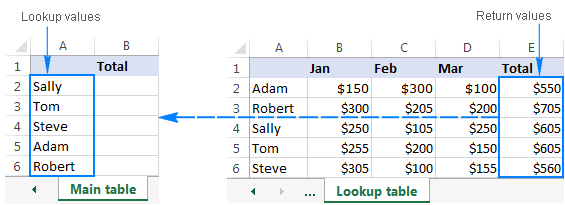## Excel Lookup functions

Below is a quick overview of the most popular formulas to perform lookup in Excel, their main advantages and drawbacks.

### LOOKUP function

The LOOKUP function in Excel can perform the simplest types of vertical and horizontal lookups.

Pros: Easy-to-use.

Cons: Limited functionality, cannot work with unsorted data (requires sorting the lookup column/row in ascending order).

### VLOOKUP function

It's an improved version of the LOOKUP function specially designed to do vertical lookup in columns.

Pros: Relatively easy to use, can work with exact and approximate match.

Cons: Cannot look at its left, stops working when a column is inserted into or removed from the lookup table, a lookup value cannot exceed 255 characters, requires much processing power on large datasets.

### HLOOKUP function

It's a horizontal counterpart of VLOOKUP that searches for a value in the first row of the lookup table and returns the value in the same position from another row.

Pros: Easy to use, can return exact and approximate matches.

Cons: Can only search in the topmost row of the lookup table, is affected by the insertion or deletion of rows, a lookup value should be under 255 characters.

### VLOOKUP MATCH / HLOOKUP MATCH

A dynamic column or row reference created by MATCH makes this Excel lookup formula immune to the changes made in the dataset. In other words, with some help from MATCH, the VLOOKUP and HLOOKUP functions can return correct values no matter how many columns/rows have been inserted to or deleted from a lookup table.

Formula for Vertical lookup

VLOOKUP(lookup_value, lookup_table, MATCH(return_column_name, column_headers, 0), FALSE)

Formula for Horizontal lookup

HLOOKUP(lookup_value, lookup_table, MATCH(return_row_name, row_headers, 0), FALSE)

Pros: An improvement over regular Hlookup and Vlookup formulas immune to data insertion or deletion.

Cons: Not very flexible, requires a specific data structure (the lookup value supplied to the MATCH function should be exactly equal to the name of the return column), cannot work with lookup values exceeding 255 characters.

### OFFSET MATCH

A more complex but a more powerful lookup formula, free of many limitations of Vlookup and Hlookup.

#### Formula for V-Lookup

OFFSET(lookup_table, MATCH(lookup_value, OFFSET(lookup_table, 0, n, ROWS(lookup_table), 1) ,0) -1, m, 1, 1)

Where:

• n - is the lookup column offset, i. e. the number of columns to move from the starting point to the lookup column.
• m - is the return column offset, i. e. the number of columns to move from the starting point to the return column.

#### Formula for H-Lookup

OFFSET(lookup_table, m, MATCH(lookup_value, OFFSET(lookup_table, n, 0, 1, COLUMNS(lookup_table)), 0) -1, 1, 1)

Where:

• n - is the lookup row offset, i. e. the number of rows to move from the starting point to the lookup row.
• m - is the return row offset, i. e. the number of rows to move from the starting point to the return row.

#### Formula for matrix lookup (by row and column)

{=OFFSET (starting_point, MATCH (vertical_lookup_value, lookup_column, 0), MATCH (horizontal_lookup_value, lookup_row, 0))}

Please pay attention that this is an array formula, which is entered by pressing Ctrl + Shift + Enter keys at the same time.

Pros: Allows performing a left-side Vlookup, an upper Hlookup and two-way lookup (by column and row values), unaffected by changes in the data set.

Cons: Complex and difficult to remember syntax.

### INDEX MATCH

It's the best way to do vertical or horizontal lookup in Excel that can replace most of the above formulas. The Index Match formula is my personal preference and I use it for almost all of my Excel lookups.

Formula for V-Lookup

INDEX (return_column, MATCH (lookup_value, lookup_column, 0))

Formula for H-Lookup

INDEX (return_row, MATCH (lookup_value, lookup_row, 0))

Formula for matrix lookup

An extension of the classic Index Match formula to return a value at the intersection of a specific column and row:

INDEX (lookup_table, MATCH (vertical_lookup_value, lookup_column, 0), MATCH (horizontal_lookup_value, lookup_row, 0))

Cons: Just one - you need to remember the formula's syntax.

Pros: The most versatile Lookup formula in Excel, superior to Vlookup, Hlookup and Lookup functions in many respects:

• It can do left and upper lookups.
• Allows safely extending or collapsing the lookup table by inserting or deleting columns and rows.
• No limit to the lookup value's size.
• Works faster. Because an Index Match formula references columns/rows rather than an entire table, it requires less processing power and won't slow down your Excel.

### Excel Lookup comparison table

As you see, not all Excel Lookup formulas are equivalent, some can handle a number of different lookups while others can only be used in a specific situation. The table below outlines the capabilities of each Lookup formula in Excel.

 Formula Vertical lookup Left lookup Horizontal lookup Upper lookup Matrix lookup Allows data insertion/deletion Lookup ✓ ✓ Vlookup ✓ Hlookup ✓ Vlookup Match ✓ ✓ Hlookup Match ✓ ✓ Offset Match ✓ ✓ ✓ ✓ ✓ Offset Match Match ✓ ✓ Index Match ✓ ✓ ✓ ✓ ✓ Index Match Match ✓ ✓

## Excel lookup formula examples

The first step in deciding which formula to use in a specific situation is to determine what kind of lookup you want to perform. Below you will find formula examples for most popular lookup types:

### Vertical lookup in columns

A vertical lookup or Vlookup is the process of finding a lookup value in one column and returning a value in the same row from another column. Vlookup in Excel can be done in a variety of ways, including:

#### VLOOKUP function

If your lookup values reside in the left hand column of the table, and you do not plan to do any structural changes to your dataset (neither add nor delete columns), you can safely use a regular Vlookup formula:

`=VLOOKUP(G2, \$A\$2:\$E\$6, 5, FALSE)`

Where G2 is the lookup value, A2:E6 in the lookup table, and E is the return column.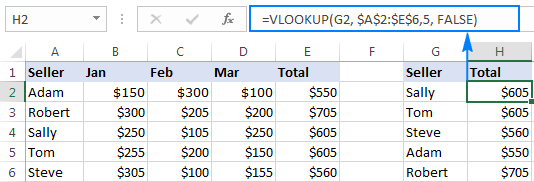#### VLOOKUP MATCH

If you are working with a "variable" Excel lookup table where columns can be inserted and deleted at any time, make your Vlookup formula immune to those changes by embedding the Match function that creates a dynamic column reference instead of a "hard-coded" index number:

`=VLOOKUP(F2,\$A\$1:\$D\$6, MATCH(\$G\$1,\$A\$1:\$D\$1, 0), FALSE)`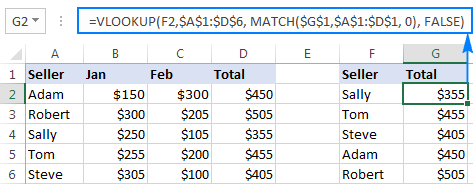#### INDEX MATCH - Left lookup

It's my favorite formula that handles right to left lookups with ease and works impeccably no matter how many columns you add or delete.

For example, to search column B for the value in H2 and return a match from column F, use this formula:

`=INDEX(\$F\$2:\$F\$6,(MATCH(H2,\$B\$2:\$B\$6,0)))`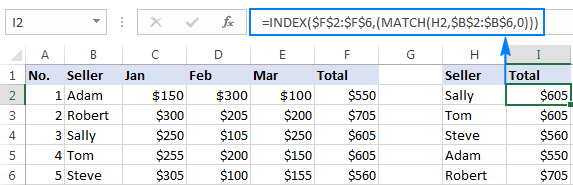Note. When you plan to use a Vlookup formula in more than one cell, you should always lock the lookup table reference by using the \$ sign (absolute cell reference), so that the formula gets copied correctly to other cells.

### Horizontal lookup in rows

A horizontal lookup is a "transposed" version of vertical lookup that searches in a horizontally arranged dataset. In other words, it searches for the lookup value in one row, and returns a value in the same position from another row.

Assuming your lookup value is in B9, lookup table is B1:F5, and you want to return a matching value from row 5, use one of the following formulas:

#### HLOOKUP function

Can look up only across the top row in your data set.

`=HLOOKUP(B8, \$B\$1:\$F\$5, 5, FALSE)`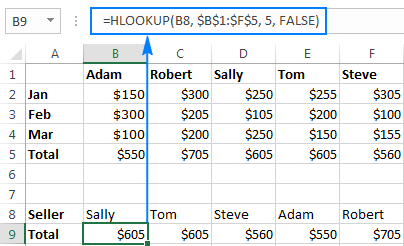#### HLOOKUP MATCH

Like pure Hlookup, this formula can search only in the topmost row, but allows you to safely insert or delete rows in the lookup table.

`=HLOOKUP(B8, \$B\$1:\$F\$5, MATCH(\$A\$9, \$A\$1:\$A\$5, 0), FALSE)`

Where A1:A5 are row headers and A9 is the name of the row from which you want to return matches.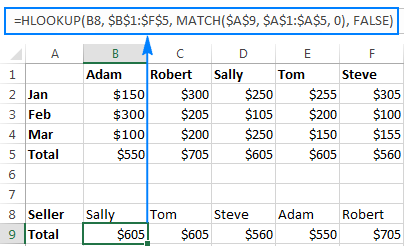#### INDEX MATCH

Can look up in any row, and has none of the limitations of the above formulas.

`=INDEX(\$B\$5:\$F\$5,(MATCH(B8,\$B\$1:\$F\$1,0)))`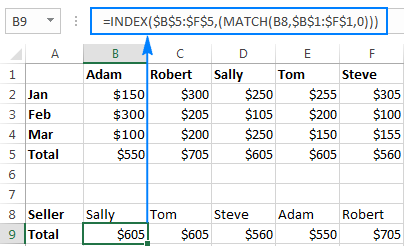### Two-dimensional lookup (based on row and column values)

Two-dimensional lookup (aka matrix lookup, double lookup or 2-way lookup) returns a value based on matches in both rows and columns. In other words, a 2-dimensional lookup formula searches for a value at the intersection of a specified row and column.

Assuming your lookup table is A1:E6, cell H2 contains the value to match on the rows and H3 holds the value to match on the columns, the following formulas will work a treat:

INDEX MATCH MATCH formula:

`=INDEX(\$A\$1:\$E\$6, MATCH(H2,\$A\$1:\$A\$6,0), MATCH(H3,\$A\$1:\$E\$1,0))`

OFFSET MATCH MATCH formula:

`=OFFSET(\$A\$1,MATCH(H2,\$A\$2:\$A\$6,0),MATCH(H3,\$B\$1:\$E\$1,0))`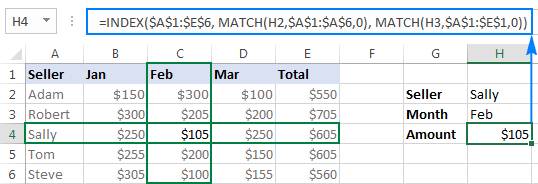Apart from the above formulas, there exist a handful of other ways to perform matrix lookup in Excel, and you can find full details in How to do 2-way lookup in Excel.

### Three-dimensional lookup

Three-dimensional lookup means searching by 3 different lookup values. In a data set below, supposing you want to search for a specific year (H2), then for a specific name within that year data (H3), and then return a value for a specific month (H4).

The task can be accomplished with the following array formula (please remember to press Ctrl + Shift + Enter to complete it correctly):

`=INDEX(\$A\$1:\$E\$12,MIN(IF((ROW(\$A\$1:\$A\$12)>MATCH(H2,\$A\$1:\$A\$12,0))*(\$A\$1:\$A\$12=H3),ROW(\$A\$1:\$A\$12),"")),MATCH(H4,\$A\$1:\$E\$1,0))`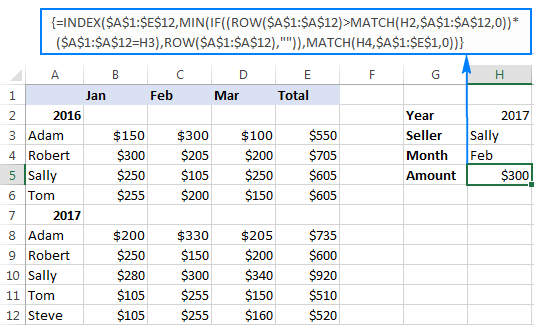### Lookup with multiple criteria

To be able to evaluate multiple criteria, we will need to modify the classic Index Match formula so that it turns into an array formula:

INDEX(lookup_table, MATCH(1, (lookup_value1=lookup_column1) * (lookup_value2=lookup_column2)*…, 0), return_column_number)

With the lookup table residing in A1:C11, let's find a match by 2 criteria: search column A for a value in cell F1, and column B for a value in cell F2:

`=INDEX(\$A\$1:\$C\$11, MATCH(1, (F1=\$A\$1:\$A\$11) * (F2=\$B\$1:\$B\$11),0), 3)`

As usual, you press Ctrl + Shift + Enter for the formula to be evaluated as an array formula.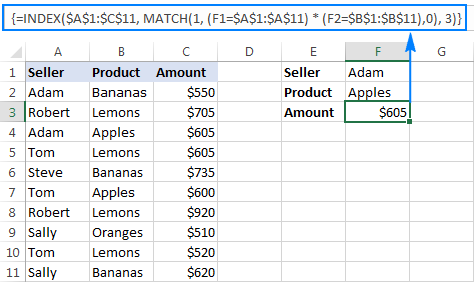For the detailed explanation of the formula's logic, please see INDEX MATCH to look up with multiple criteria.

### Lookup to return multiple values

Whichever Excel Lookup function you use (LOOKUP, VLOOKUP, or HLOOKUP), it can only return a single match. To get all found matches, you would have to employ 6 different functions combined in an array formula:

IFERROR(INDEX( return_range, SMALL(IF( lookup_value=lookup_range, ROW( return_range )- m ,""), ROW() - n )),"")

Where:

• m is the row number of the first cell in the return range minus 1.
• n is the row number of the first formula cell minus 1.

With the lookup value located in cell E2, lookup range in A2:A11, return range in B2:B11, and the first formula cell in row 2, your lookup formula takes the following shape:

`=IFERROR(INDEX(\$B\$2:\$B\$11, SMALL(IF(\$E\$2 =\$A\$2:\$A\$11, ROW(\$B\$2:\$B\$11 )- 1,""), ROW() - 1 )),"")`

For the formula to return multiple matches, you enter it in the first cell (F2), press Ctrl + Shift + Enter, and then copy the formula to other cells down the column.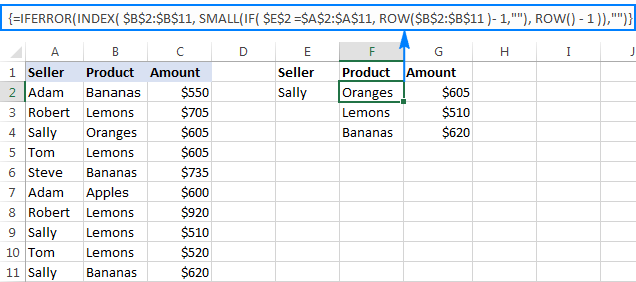For the detailed explanation of the above formula and other ways to return multiple values, please see How to Vlookup to return multiple results.

### Nested lookup (from 2 lookup tables)

In situations when your main table and the lookup table from which you want to pull data do not have a common column, you can use an additional lookup table to establish matches, like this: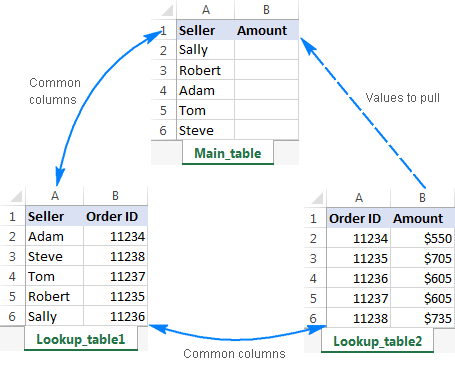To retrieve the values from the Amount column in Lookup_table2, you use the following formula:

`=VLOOKUP(VLOOKUP(A2, Lookup_table1!\$A\$1:\$B\$6, 2, FALSE), Lookup_table2!\$A\$1:\$B\$6, 2, FALSE)`

As shown in the screenshot below, our nested lookup formula works perfectly: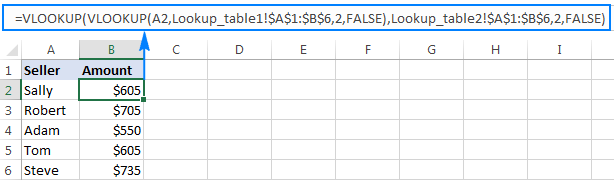### Sequential Vlookups from multiple sheets

To perform sequential Vlookups based on whether a previous lookup succeed or failed, use nested IFERROR functions together with VLOOKUPs to evaluate multiple conditions one by one:

If the first Vlookup fails, IFERROR traps the error and runs another Vlookup. If the second Vlookup does not find anything either, the second IFERROR catches the error and runs the third Vlookup, and so on. If all Vlookups fail, the last IFERROR returns "not found" or any other message you supply to the formula.

As an example, let's try to pull the amount from 3 different sheets:

`=IFERROR(VLOOKUP(B1,A6:B9,2,0), IFERROR(VLOOKUP(B1,D6:E9,2,0), IFERROR(VLOOKUP(B1,G6:H9,2,0), "Not found")))`

The result will look something similar to this: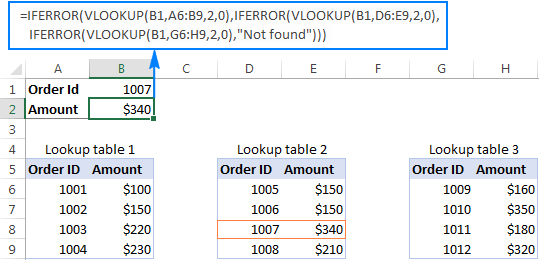### Case-sensitive lookup

As you probably know, all Excel Lookup functions are case-insensitive by their nature. To force your lookup formula to distinguish between lowercase and uppercase text, use either LOOKUP or INDEX MATCH in combination with the EXACT function. I personally opt for INDEX MATCH because it does not require sorting values in the lookup column as the LOOKUP function does, can perform both left-to-right and right-to-left lookups, and works perfectly for all data types.

INDEX(return_column, MATCH(TRUE, EXACT(lookup_column, lookup_value),0))

With G2 being the lookup value, A - column to look up against and E - column to return the matches from, our case-sensitive lookup formula goes as follows:

`=INDEX(\$E\$2:\$E\$6, MATCH(TRUE, EXACT(\$A\$2:\$A\$6,G2),0))`

Since it's an array formula, be sure to press Ctrl + Shift + Enter to complete it properly.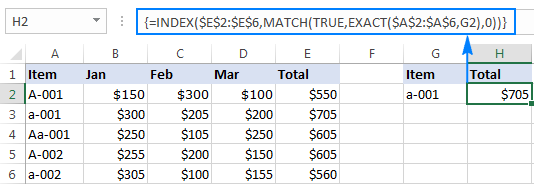For more formula examples, please see How to do case-sensitive lookup in Excel.

### Lookup partial string match

Looking up by partial match is one of the most challenging tasks in Excel for which there exists no universal solution. Which formula to use depends on what kind of differences there are between your lookup values and values in the column to search in. In most cases, you'd use the LEFT, RIGHT or MID function to extract the common part of the values, and then supply that part to the lookup_value argument of the Vlookup function like it's done in the following formula:

`=VLOOKUP(RIGHT(D2,4), \$A\$2:\$B\$6, 2, FALSE)`

Where D2 is the lookup value, A2:B6 is the lookup table and 2 in the index number of the column to return the matches from.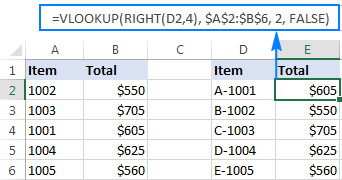For other ways to perform a partial match lookup in Excel, please see How to merge two worksheets by partial match.

This is how you use the Lookup functions in Excel. To have a closer look at the formulas discussed in this tutorial, you are welcome to download our Excel Lookup formula examples.

## Formula-free way to do lookup in Excel

It goes without saying that Excel lookup is not a trivial task. If you are taking your first steps in learning the realm of Excel, lookup formulas may seem quite confusing and difficult to understand. But please, don't feel discouraged, these skills do not come naturally to the majority of users!

To make things easier for novices, we created a special tool, Merge Tables Wizard, that can look up, match and merge tables without a single formula. In addition, it provides a number of really unique options that even advanced Excel users can benefit from:

• Lookup by multiple criteria, i.e. use one or several columns as the unique identifier(s).
• Update values in existing columns and add new columns from the lookup table.
• Return multiple matches in separate rows. When used in combination with the Combine Rows Wizard, it can even return multiple results in a single cell, comma or otherwise separated (an example can be found here).
• And more.

Working with the Merge Tables Wizard is easy and intuitive. All you have to do is:

1. Select your main table where you want to pull matching values.
2. Select the lookup table to pull the matches from.
3. Define one or more common columns.
4. Choose the columns to be updated or/and added to the end of the table.
5. Optionally, select one or more additional merge options.
6. Click Finish and you will have a result in a moment!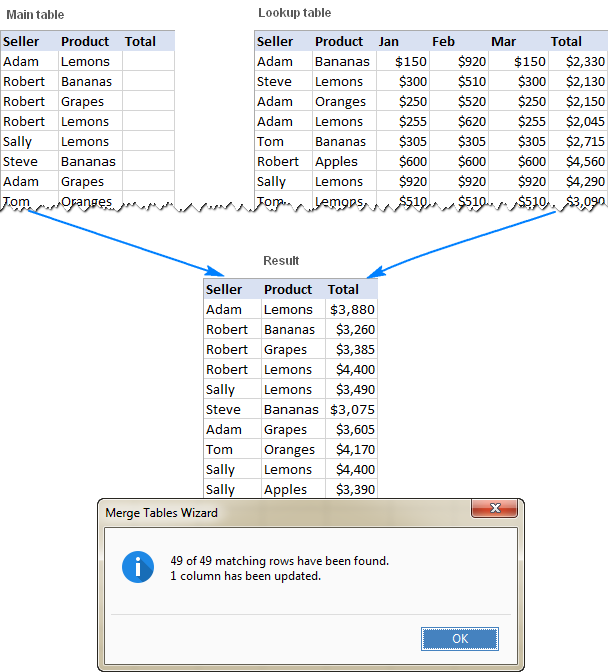If you are curious to try the add-in on your own worksheets, you are welcome to download a trial version of our Ultimate Suite that includes all our time-saving tools for Excel (in total, 70+ tools and 300+ features!).

Excel Lookup formula examples (.xlsx file)
Ultimate Suite 14-day fully-functional version (.exe file)

## You may also be interested in

1. Hi
I have a list of 100 employees and the salary paid over 5 years for every month (60 columns). I need to get the salary numbers updated in another sheet with the help of vlookup (lookup value is name of the individual)

I am able to input formulas for a few rows but to extend it to all the 100 names and 60 columns, I am finding it difficult. Can you please help?

• Hi!
You can get a list of salaries for the employee you specify by using the FILTER formula. For more information, read this article: Excel FILTER function - dynamic filtering with formulas.
I hope it’ll be helpful. If this is not what you wanted, please describe the problem in more detail.

• Hi Alexandar

Thank you for your quick response.
I have raw data of 1000 employees' salary details paid over 60 months (in 60 columns, each column representing each month). I need to update in a certain format with only 400 employees out of 1000 along with the 60 months' salary given each month (in a Row)
Jan 20 Feb20 Mar20. Apr20 May20. Jun20 .....
Adam 2345 2320. 2450. 2345. 3135. 3152.........

• Hi!
If I understand correctly, you can use the FILTER function as I recommended earlier. For example,

If that doesn't work for you, explain in detail what result you want to get and how you want to select the 400 employees

2. excellent formulas i can work very quickly through them

3. Being vehicle sold |MAT55345GDG45|
Being vehicle spares sold|MAT45789TYF67|

I want to extract text starting with MAT

4. What has me confused is why THE FORMULA. = lookup ( Q14 , ' / Volumes / master links etc

WHY IS Volumes used ?

5. Hello,
Is there a way that excel identify a cell which contain any kind of dictionary words ?

• Hello Saeid!
You can use conditional formatting to select cells with the right words. In the condition formula, you can use the VLOOKUP function. Read more about this on our blog here and here.

6. HI,
WE ARE LOOKING YOUR FORMULA'S VERY USEFUL AND VERY SHORT CUT WAY IN EXCEL PLEASE THANK YOU VERY VERY MUCH
DEAR
SHARATH

7. Hi Svetlana,
I believe you are the only person who can help me with my problem. I have a 2D text table and I would like to pick out the most common text string, the second most common text string, the third and so on. Can that be done?

For example, my table is like this:
A B C D E
B B C D E
B A A F G

My formula should search the above and return 'B', 'A', ...

Thank you in anticipation.

8. I got a challenge for you or whomever wants to try this.

Subject: select all text after an occurrence of a phrase in that cell looked up in a Range of phrases to see if it's present.

I have a bunch of 'employer updates' text copied from a website in Sheet 1, Col. A, with each row containing 1 person's data.

The first part of each cell is ALWAYS the person's name followed by a space then a "(".

The gradation year is ALWAYS after that ( and formatted as: '##,

After the comma is the person's college major.

Ex: John Smith ('91, Accounting) was promoted to...

I already have my formulas to extract the name (cell B2)...

=TRIM(LEFT(A2,FIND("(",A2,1)-2))

=TRIM(MID(A2,FIND("'",A2,1)+1,2))

but now I need their Position/Title in cell D2 (and for each row thereafter). The position/title is usually prefaced by an introductory phrase like:

has a new position as
has been named
has joined
is now a
is/has been promoted to
was named

I had originally put that block of phrase data in cells J2-J7 and selected them all to define them as Range "TITLE".

I thought I could use a formula to extract all text in cell A2 coming AFTER any of those phrases if found in the Range TITLE to create col. D with their position/title.

I can't figure out a way to do this. Am I going about it the wrong way? Is there an easier solution I am missing?

Regards,
Ky

9. I want a formula in column "B" that can allocate one sequential serial no. to all in-sequential duplicates figures in a column "A"

"A" "B"
0 1
15 5
5 4
3 3
0 1
3 3
1 2

10. I have to manage increase salaries to employees for my organization. Need to change their pay in 2017 with respect to their previous pay in 2016 comparing with BPS. please help me in emailing you my excel document

11. i have invoice worksheet n sales worksheet when i enter data in sales worksheet it get updated on invoice worksheet and i can print the invoice directly but i am not able to add more then 1 product lines in 1 invoice.. please help what formula do i need to use to more then one product in invoice

• Hi, Amit,

the point is that Excel lookup functions don't allow working with the tons of data at the same time. As a rule, the formula should be copied to every cell where you want to see your new value.

However, we have a great tool that can update a whole worksheet with the data from your lookup table in a couple of clicks. Pleas take a look at our Merge Tables Wizard. You can download its fully functional trial version and try it out for yourself.

Hope this helps!

12. Hi,

Firstly, apologies if this has been answered in another blog post previously!

I have an Excel spreadsheet which is essentially a 'call schedule' with around 1,000 rows of client details,last contact dates, future contact dates (Column A) contact notes (Column N), etc.

Column A11 - A1000 has the next 'due date' - there are unique and duplicate dates right throughout this column. Column D has the Client Name. Column N has the contact note (what I want to talk with people about) that I have set.

What I would like to do is have the next 5 due dates show in order in cells D2 -D7, and then display the corresponding client name (E2 - E7) and contact note (F2 - F7).

I have used the formula =small(\$A\$11:\$A:\$1000,1) in D2 and then in E2 the formula =VLOOKUP(D2,\$A\$11:\$N\$1000,4,false) etc.

The issue is that 'Vlookup' will only bring back the first response - if the date 02 June is the first 3 results for the 'small' function for example, it will show the same client name three times rather than the next... I believe it needs an 'index' and Array function, but that's beyond my excel knowledge!

Anyone able to help?? Would greatly appreciate any assistance! Cheers.

• Can you just sort the table by the due date? Newest to oldest

13. Lots of thanks, for your cooperation, Svetlana.

14. Hi, Svetlana, Thanks for your post. I don't understand the Three dimensional lookup formula example (=INDEX(\$A\$1:\$E\$12,MIN(IF((ROW(\$A\$1:\$A\$12)>MATCH(H2,\$A\$1:\$A\$12,0))*(\$A\$1:\$A\$12=H3),ROW(\$A\$1:\$A\$12),"")),MATCH(H4,\$A\$1:\$E\$1,0))). Would you please explain it for my perusal?

• Hi Imran,

In truth, it is one of those formulas that are easier to write than explain :) Nevertheless, I will do my best to describe the general logic.

At the core, you use the IF statement to find the number of the "Seller" (H3) row that comes after the "Year" (H2) row. Here's how:

MATCH(H2,\$A\$1:\$A\$12,0) returns the row number of the year of interest, which is 7 (A7) in this example.

(ROW(\$A\$1:\$A\$12)>MATCH(H2,\$A\$1:\$A\$12,0)) compares each row in the table (A1:A12) with the above number and returns TRUE only for rows greater than 7, FALSE otherwise.

(\$A\$1:\$A\$12=\$H\$3) compares the values in column A with the seller name in H3, and returns TRUE for the matches, FALSE otherwise.

Then, you multiply the above 2 arrays of TRUE's and FALSE's. Remembering that TRUE equates to 1 and FALSE to 0, and multiplying by 0 always gives 0, you get 1 only for those rows that are after row 7 (the year 2017) and where the seller name matches the name in H3 (Sally in our example). For such rows, you get the IF function to return the row number (by putting ROW(\$A\$1:\$A\$12) in the value_if_true argument), an empty string ("") otherwise.

Since there may be a few occurrences of the same name in column A, you use the MIN function to get the first row number containing the desired name (that comes after the desired year).

The rest of the formula is easy. You supply the above row number in the row_number argument of the INDEX function. To identify the column, you put MATCH(H4,\$A\$1:\$E\$1,0) in the column_number argument, where H4 is the month for which you want to return the amount. That's it!

15. One of the Best Post.

Thanks for sharing ☺..........